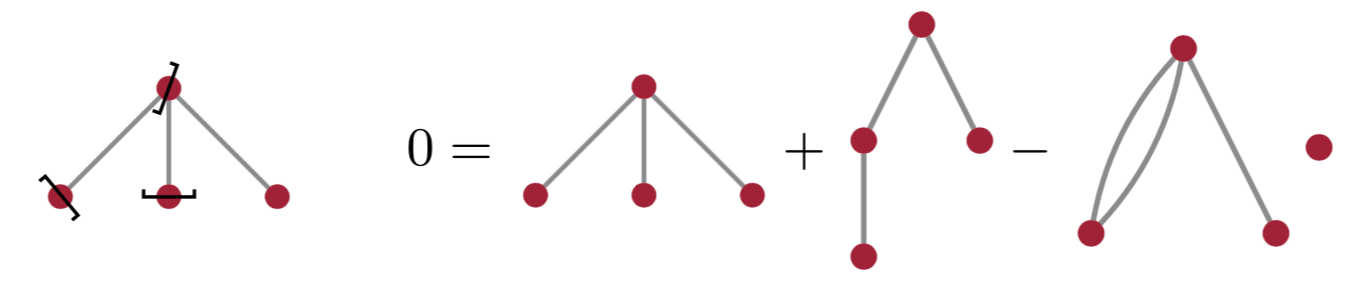# Cutting Multiparticle Correlators Down to Size### Abstract

Multiparticle correlators are mathematical objects frequently encountered in quantum field theory and collider physics. By translating multiparticle correlators into the language of graph theory, we can gain new insights into their structure as well as identify efficient ways to manipulate them. In this paper, we highlight the power of this graph-theoretic approach by “cutting open” the vertices and edges of the graphs, allowing us to systematically classify linear relations among multiparticle correlators and develop faster methods for their computation. The naive computational complexity of an $N$-point correlator among $M$ particles is $O(M^N)$, but when the pairwise distances between particles can be cast as an inner product, we show that all such correlators can be computed in linear $O(M)$ runtime. With the help of new tensorial objects called Energy Flow Moments, we achieve a fast implementation of jet substructure observables like $C_2$ and $D_2$, which are widely used at the Large Hadron Collider to identify boosted hadronic resonances. As another application, we compute the number of leafless multigraphs with $d$ edges up to $d = 16$ (15,641,159), conjecturing that this is the same as the number of independent kinematic polynomials of degree $d$, previously known only to $d=8$ (279).

Type
Publication
Physical Review D, 101 036019
Date